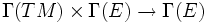# Affine space of connections

This article defines a basic construct that makes sense on any differential manifold
View a complete list of basic constructs on differential manifolds

## Definition

Given a differential manifold$M$ and a vector bundle$E$ over$M$, the affine space of connections on$M$ is defined as the set of all connections on$M$, viewed as a subset of the vector space of all bilinear maps:$\Gamma(TM) \times \Gamma(E) \to \Gamma(E)$

This subset is in fact an affine space, because the condition of being a difference of two connections is a linear system of conditions. Fill this in later